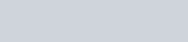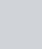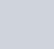A game of chance consists of spinning an arrow which is equally
Question:

A game of chance consists of spinning an arrow which is equally likely to come to rest pointing to one of the number, 1, 2, 3, …, 12 as shown in following figure. What is the probability that it will point to:(i) 10?

(ii) an odd number?

(iii) a number which is multiple of 3?

(iv) an even number?

Solution:

GIVEN: A game of chance consists of spinning an arrow which is equally likely to come to rest pointing number 1,2,3…..12

TO FIND: Probability of following

Total number on the spin is 12

(i) Favorable event i.e. to get 10 is 1

Total number of Favorable event i.e. to get 10 is 1

We know that PROBABILITY =Hence probability of getting a10(ii) Favorable event i.e. to get an odd number are 1,3,5,7,9,11,

Total number of Favorable event i.e. to get a prime number is 6

We know that PROBABILITY =Hence probability of getting a prime number is(iii) Favorable event i.e. to get an multiple of 3 are 3,6,9,12

Total number of Favorable event i.e. to get a multiple of 3 is 4

We know that PROBABILITY =Hence probability of getting multiple of 3 =(iv) Favorable event i.e. to get an even number are 2,4,6,8,10,12

Total number of Favorable events i.e. to get an even number is 6

We know that PROBABILITY =Hence probability of getting an even number is